# The 2018-2019 Annual Appeal

Northside needs your help. We are a nationally recognized high school, and the funds we receive from CPS do not support the necessary work the administration, staff and teachers put in to ensure our kids receive the proper education.

How does your donation to the Annual Appeal benefit Northside?

▪ Staffing for college counseling
▪ Technology support
▪ Uniforms and equipment for our athletic teams
▪ Wellness Center support
▪ School security
▪ Transportation for field trips
▪ Funding for student clubs
▪ New copiers for the school
▪ Professional development for teachers
 ▪ Staffing for college counseling
 ▪ Technology support
 ▪ Uniforms and equipment for our athletic teams
 ▪ Wellness Center support
 ▪ School security
 ▪ Transportation for field trips
 ▪ Funding for student clubs
 ▪ New copiers for the school
 ▪ Professional development for teachers

Friends of Northside purchased all of these items with funds raised by the Annual Appeal. The Annual Appeal raises the money that maintains continuity of programming and personnel in our school, allowing Northside to build its strengths year upon year, rather than trying to make do with gaps created by budget cuts.

Your donations are critical in keeping Northside the nationally recognized high school that it is and always deserves to be.

Keep Northside Strong! All donations are greatly appreciated!

Click HERE (English) or HERE (Español) to read the appeal letter from Principal Mest.

Questions? Click HERE to contact Friends of Northside.

 table div table+table+table+table div table{width:100%;padding:0}table div table+table+table+table div table img{width:96.23%;padding:0;float:none}table div table+table+table+table div table td{width:100%;padding:0 1.88% 18px}/* styles */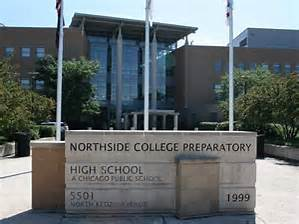# Northside's Selective Enrollment Open House

## Student and Parent Volunteers Needed

Saturday, October 20
11:00 am - 2:00 pm

Please encourage your students to sign up to help with the Open House. Student volunteers are needed from 9:00 am - 3:00 pm. There will be 2 training sessions: October 3 and 17. Students will be able to pick from X or Y sessions. On the day of the Open House, students will show families around, answer questions, and have the opportunity to share what they love about Northside. Pizza and snacks will be provided and students will earn service learning hours. Please encourage students to fill out this FORM to sign up.

We also need 100 adult volunteers to work this busy but super-fun event! Parents help answer questions and direct crowds in the gym, outside and all over the building. You will need to be registered as a Level II volunteer if you have not done so already. It is very easy to do. Please see details below under CPS Volunteer Requirements.

Sign up HERE to donate snacks for the students.

 table div table+table+table+table+table+table+table div table{width:100%;padding:0}table div table+table+table+table+table+table+table div table img{width:96.23%;padding:0;float:none}table div table+table+table+table+table+table+table div table td{width:100%;padding:0 1.88% 18px}/* styles */# Parking Lot Safety

Parents, please remember that ALL VEHICLES MUST EXIT ONTO KEDZIE. You may enter the parking lot from Kedzie or Bryn Mawr, but regardless of where you enter, all cars must exit onto Kedzie. For the safety of our students and the rest of the Northside community, please do not exit onto Bryn Mawr.

 table div table+table+table+table+table+table+table+table+table div table{width:100%;padding:0}table div table+table+table+table+table+table+table+table+table div table img{width:96.23%;padding:0;float:none}table div table+table+table+table+table+table+table+table+table div table td{width:100%;padding:0 1.88% 18px}/* styles *//* styles */ Friends of Northside asks every family to contribute 5 hours to Northside each year. This could include staffing school events, chaperoning field trips, assisting with the Gala and more.# Halloween Dance

Friday, October 26

Please volunteer to chaperone this annual dance. Students love it and without our parent chaperones, it wouldn't be possible. All volunteers must be CPS Level II Volunteer certified. Sign up HERE.

# Volunteers Still Needed

Make a commitment to Northside by offering your time to work with other volunteers in one of these rewarding, on-going positions. Email Friends of Northside to indicate your interest or ask questions.

## Eblast Co-Editor

Compose and distribute This Week at Northside and prepare other standalone blasts as requested.

## Facebook/Social Media Editor

Update and maintain the Friends of Northside Facebook and Twitter accounts.

## Graphic Designer

Provide professional guidance and materials for various design needs throughout the year.

## Website Editor

Keep the Friends of Northside website current and enhance its visual appeal.

## Database Manager

Maintain the Friends of Northside CRM (customer relationship management) database and develop meaningful reports.

## Join the Spring Benefit Committee

The Benefit is NCP’s major spring fundraiser. Get involved, get to know NCP staff and parents and make a profound impact on Northside College Prep.

When is the Spring Benefit ?
Friday, March 8, 2019

What’s the time commitment for the team?
Meetings begin at the end of September. The time commitment depends on your role. There is something for everyone. Please join us!

What roles are available?
* Entertainment Chair - one or two people to determine and arrange the entertainment for the Gala.
* Auction Item Solicitors - at least five people to solicit auction items. It requires just a few hours a week and can be done in person or via phone or email.

Email ncpgaladonate@gmail.com for more information or to join the team!

 table div table+table+table+table+table+table+table+table+table+table+table+table+table+table+table div table{width:100%;padding:0}table div table+table+table+table+table+table+table+table+table+table+table+table+table+table+table div table img{width:96.23%;padding:0;float:none}table div table+table+table+table+table+table+table+table+table+table+table+table+table+table+table div table td{width:100%;padding:0 1.88% 18px}/* styles */# CPS Volunteer Requirements

According to CPS policy, anybody who is going to be in contact with students must be approved as a CPS volunteer. There are two levels of volunteers, Level 1 and Level 2.

## Level II Volunteers

(This process can be completed in a day & is required even of volunteers for Friends of Northside events)
A fingerprint criminal background check is not required for Level II Volunteers.

A Level II Volunteer is:

1. A parent providing supervised volunteer service in their child’s school or classroom for less than 10 hours/week;
2. Any individual providing volunteer service for less than 5 hours/week at a school where s/he does not have a child enrolled;
3. An individual providing incidental volunteer service with no ongoing individualized interaction with a student(s) including those who speak at a class/assembly, judge academic competitions, give musical performance, participate in the “Principal for a Day” program, job shadowing event or other one-time event provided where there is direct supervision of the activity/event by regular school employees;
4. A parent accompanying his/her child’s class on a one-day field trip or another type of extracurricular activity that does not involve an overnight stay;
5. An individual providing volunteer service on projects/activities involving no or nominal contact with children (home-based volunteer activities, volunteers serving at Central or Network office).
 1 A parent providing supervised volunteer service in their child’s school or classroom for less than 10 hours/week;
 2 Any individual providing volunteer service for less than 5 hours/week at a school where s/he does not have a child enrolled;
 3 An individual providing incidental volunteer service with no ongoing individualized interaction with a student(s) including those who speak at a class/assembly, judge academic competitions, give musical performance, participate in the “Principal for a Day” program, job shadowing event or other one-time event provided where there is direct supervision of the activity/event by regular school employees;
 4 A parent accompanying his/her child’s class on a one-day field trip or another type of extracurricular activity that does not involve an overnight stay;
 5 An individual providing volunteer service on projects/activities involving no or nominal contact with children (home-based volunteer activities, volunteers serving at Central or Network office).

## Level I Volunteers

(Please allow 8-12 weeks for the entire process)
A fingerprint criminal background check is required for all Level I Volunteers.

A Level I volunteer is:

1. A parent providing volunteer service in their child’s school or classroom for 10 or more hours/week;
2. An individual providing volunteer service for 5 or more hours/week at a school where s/he does not have a child enrolled;
3. Any individual chaperoning an overnight school-sponsored trip, regardless of the hours/week that the volunteer serves (See also the Student Travel Policy).
 1 A parent providing volunteer service in their child’s school or classroom for 10 or more hours/week;
 2 An individual providing volunteer service for 5 or more hours/week at a school where s/he does not have a child enrolled;
 3 Any individual chaperoning an overnight school-sponsored trip, regardless of the hours/week that the volunteer serves (See also the Student Travel Policy).

To become a volunteer, please visit CPS' Volunteer website and follow the instructions. There is also a link on Northside's website on the Parents page Northside Volunteers.

Assistant Principal Kaitlin O'Hare is Northside's Volunteer Coordinator. After applying to become a volunteer, you must visit the school and show Ms. O'Hare your ID, so she can approve you to move forward in the volunteer process. For Level II volunteers, that is the only step you must take. For Level 1, you will receive further instructions from CPS.

 table div table+table+table+table+table+table+table+table+table+table+table+table+table+table+table+table+table div table{width:100%;padding:0}table div table+table+table+table+table+table+table+table+table+table+table+table+table+table+table+table+table div table img{width:96.23%;padding:0;float:none}table div table+table+table+table+table+table+table+table+table+table+table+table+table+table+table+table+table div table td{width:100%;padding:0 1.88% 18px}/* styles */# This Week in Sports

Come to a game or match and support our student athletes!

## Men's Soccer

▪ Wednesday, October 10, 4:30 (V) and 6:15 (JV) pm vs Latin at NCP
▪ Saturday, October 13, details TBD (SO)
 ▪ Wednesday, October 10, 4:30 (V) and 6:15 (JV) pm vs Latin at NCP
 ▪ Saturday, October 13, details TBD (SO)

## Women's Volleyball

▪ Tuesday, October 9, 5:00 (V) and 6:00 (SO) pm vs Back of the Yards HS - CPS playoffs at NCP
 ▪ Tuesday, October 9, 5:00 (V) and 6:00 (SO) pm vs Back of the Yards HS - CPS playoffs at NCP
 /* styles */ Visit the NCP Athletics website for additional information about Northside teams, and download the 8 to 18 app via iTunes or Android to follow them on your phone.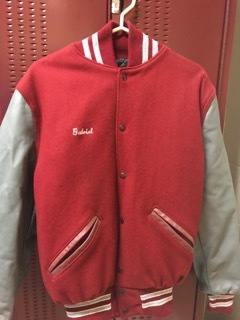# Order Your Northside Athletic Jacket Now

If you're interested in purchasing an athletic jacket, please see Mr. Pagani in the gym for an order form or click HERE to download a copy.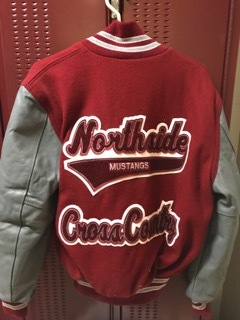Contact Mr. Pagani with any questions.

Deadline to order: Wednesday, October 10

 table div table+table+table+table+table+table+table+table+table+table+table+table+table+table+table+table+table+table+table+table+table+table div table{width:100%;padding:0}table div table+table+table+table+table+table+table+table+table+table+table+table+table+table+table+table+table+table+table+table+table+table div table img{width:96.23%;padding:0;float:none}table div table+table+table+table+table+table+table+table+table+table+table+table+table+table+table+table+table+table+table+table+table+table div table td{width:100%;padding:0 1.88% 18px}/* styles */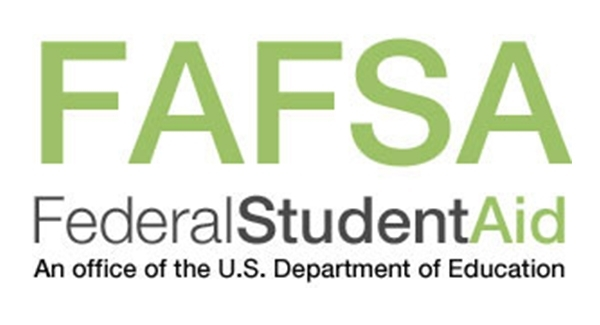# FAFSA Completion Workshop

## Presented by Ladder Up

Tuesday, October 16
6:00 - 8:00 pm
NCP Library, 2nd Floor

The FAFSA form should be filled out as soon as possible because it helps to determine your eligibility for federal, state, local and college scholarships, grants and loans. If you have not completed your FAFSA form or have questions, this workshop will provide students and parents support with their financial aid forms.

For students attending college in the 2019-2020 school year, you will need financial information from 2017.

· Your Social Security card. It is important that you enter your Social Security Number correctly!

· Your (and if married, your spouse's) 2017 federal income tax return and any other records so that you can prepare your FAFSA carefully and correctly

Contact Mr. Alexander Hughes in the counseling office if you have any questions. He can be reached at 773-534-3974 or ACHughes2@cps.edu.

 table div table+table+table+table+table+table+table+table+table+table+table+table+table+table+table+table+table+table+table+table+table+table+table+table div table{width:100%;padding:0}table div table+table+table+table+table+table+table+table+table+table+table+table+table+table+table+table+table+table+table+table+table+table+table+table div table img{width:96.23%;padding:0;float:none}table div table+table+table+table+table+table+table+table+table+table+table+table+table+table+table+table+table+table+table+table+table+table+table+table div table td{width:100%;padding:0 1.88% 18px}/* styles */# No School

Columbus Day
Monday, October 8

 table div table+table+table+table+table+table+table+table+table+table+table+table+table+table+table+table+table+table+table+table+table+table+table+table+table+table div table{width:100%;padding:0}table div table+table+table+table+table+table+table+table+table+table+table+table+table+table+table+table+table+table+table+table+table+table+table+table+table+table div table img{width:96.23%;padding:0;float:none}table div table+table+table+table+table+table+table+table+table+table+table+table+table+table+table+table+table+table+table+table+table+table+table+table+table+table div table td{width:100%;padding:0 1.88% 18px}/* styles */# Other Important October Dates

Monday, October 8--Columbus Day, no school
Thursday, October 11--Athletic Boosters Meeting
Tuesday, October 16--LSC Meeting
Tuesday, October 16--FAFSA Completion Workshop
Saturday, October 20--NCP Open House
Tuesday, October 23--FON Meeting
Thursday, October 25--Halloween Concert
Friday, October 26--Halloween Dance sponsored by the Latin Club

 table div table+table+table+table+table+table+table+table+table+table+table+table+table+table+table+table+table+table+table+table+table+table+table+table+table+table+table+table div table{width:100%;padding:0}table div table+table+table+table+table+table+table+table+table+table+table+table+table+table+table+table+table+table+table+table+table+table+table+table+table+table+table+table div table img{width:96.23%;padding:0;float:none}table div table+table+table+table+table+table+table+table+table+table+table+table+table+table+table+table+table+table+table+table+table+table+table+table+table+table+table+table div table td{width:100%;padding:0 1.88% 18px}/* styles */# Alumni Parent College Panel

Wednesday, November 7
6:30 - 8:00 pm
2nd Floor Atrium

You are invited to attend the annual parent panel about the college application process. Hear from parents of recent NCP alumni who will share their experiences and words of wisdom regarding their college search. Please RSVP HERE.

 table div table+table+table+table+table+table+table+table+table+table+table+table+table+table+table+table+table+table+table+table+table+table+table+table+table+table+table+table+table+table div table{width:100%;padding:0}table div table+table+table+table+table+table+table+table+table+table+table+table+table+table+table+table+table+table+table+table+table+table+table+table+table+table+table+table+table+table div table img{width:96.23%;padding:0;float:none}table div table+table+table+table+table+table+table+table+table+table+table+table+table+table+table+table+table+table+table+table+table+table+table+table+table+table+table+table+table+table div table td{width:100%;padding:0 1.88% 18px}/* styles */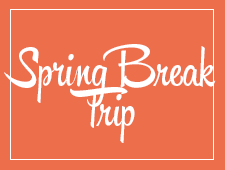# Travel this Spring with Northside

## Spring Break 2019 trip to England

On this trip you will experience the distinct character that is London. Admire St Paul's Cathedral, Big Ben, The House of Parliament, and Buckingham Palace. We will also travel to Somerset and visit Stonehenge the United Kingdom's most mysterious landmark, Bath, Stratford (see where William Shakespeare was born) and Oxford.

See Ms. Fernandez or Ms Vosnos or click HERE for an informational brochure

## Spring Break 2019 trip to the Grand Canyon

Students will have a chance to connect with the majesty of our National Park system. We will spend 5 nights in the Grand Canyon camping, hiking, stargazing and learning wilderness skills. Students will participate in a second-semester colloquium to prepare them for the trip. Trip sponsors and chaperones include Ms. Qazi, Ms. Schaldenbrand and Mr. Barry Smith. Approximate cost \$1,000 (includes flight, food, and lodging)

 table div table+table+table+table+table+table+table+table+table+table+table+table+table+table+table+table+table+table+table+table+table+table+table+table+table+table+table+table+table+table+table+table div table{width:100%;padding:0}table div table+table+table+table+table+table+table+table+table+table+table+table+table+table+table+table+table+table+table+table+table+table+table+table+table+table+table+table+table+table+table+table div table img{width:96.23%;padding:0;float:none}table div table+table+table+table+table+table+table+table+table+table+table+table+table+table+table+table+table+table+table+table+table+table+table+table+table+table+table+table+table+table+table+table div table td{width:100%;padding:0 1.88% 18px}/* styles */table.module-32{width:94.34%;padding:0}table div table+table+table+table+table+table+table+table+table+table+table+table+table+table+table+table+table+table+table+table+table+table+table+table+table+table+table+table+table+table+table+table+table div table{width:94.34%;float:none;margin-left:auto;margin-right:auto;padding:0}table div table+table+table+table+table+table+table+table+table+table+table+table+table+table+table+table+table+table+table+table+table+table+table+table+table+table+table+table+table+table+table+table+table div table a{border:0 none;text-decoration:none}table div table+table+table+table+table+table+table+table+table+table+table+table+table+table+table+table+table+table+table+table+table+table+table+table+table+table+table+table+table+table+table+table+table div table img{width:100%!important;border:0 none;text-decoration:none}table div table+table+table+table+table+table+table+table+table+table+table+table+table+table+table+table+table+table+table+table+table+table+table+table+table+table+table+table+table+table+table+table+table div table td{width:100%;padding:0}/* styles */
 /* styles */ Check out The HoofBeat, Northside's online newspaper, to get NCP news from the student perspective!
 table div table+table+table+table+table+table+table+table+table+table+table+table+table+table+table+table+table+table+table+table+table+table+table+table+table+table+table+table+table+table+table+table+table+table+table div table{width:100%;padding:0}table div table+table+table+table+table+table+table+table+table+table+table+table+table+table+table+table+table+table+table+table+table+table+table+table+table+table+table+table+table+table+table+table+table+table+table div table img{width:96.23%;padding:0;float:none}table div table+table+table+table+table+table+table+table+table+table+table+table+table+table+table+table+table+table+table+table+table+table+table+table+table+table+table+table+table+table+table+table+table+table+table div table td{width:100%;padding:0 1.88% 18px}/* styles */table.module-35{width:57.17%;padding:0}table div table+table+table+table+table+table+table+table+table+table+table+table+table+table+table+table+table+table+table+table+table+table+table+table+table+table+table+table+table+table+table+table+table+table+table+table div table{width:57.17%;float:none;margin-left:auto;margin-right:auto;padding:0}table div table+table+table+table+table+table+table+table+table+table+table+table+table+table+table+table+table+table+table+table+table+table+table+table+table+table+table+table+table+table+table+table+table+table+table+table div table a{border:0 none;text-decoration:none}table div table+table+table+table+table+table+table+table+table+table+table+table+table+table+table+table+table+table+table+table+table+table+table+table+table+table+table+table+table+table+table+table+table+table+table+table div table img{width:100%!important;border:0 none;text-decoration:none}table div table+table+table+table+table+table+table+table+table+table+table+table+table+table+table+table+table+table+table+table+table+table+table+table+table+table+table+table+table+table+table+table+table+table+table+table div table td{width:100%;padding:0}/* styles */

# What is FAN?

FAN is Family Action Network, an organization which connects parents, educators and professionals through collaborative programming that educates, inspires and positively impacts the broader community. Nearly every week during the school year there will be a dynamic and important speaker, discussing the issues that interest you.

FAN events are free to all.

# Comic Book Lessons That Shape How I Lead

Astro Teller, Ph.D.
Captain of Moonshots at X: The Moonshot Factory, the R&D lab of Alphabet, and Distignuished Alumni Award recipient, ETHS Class of 1988; Co-Founder, Cerebellum Capital

Monday, October 8, 7:00 pm
Evanston Township High School Auditorium
1600 Dodge Ave., Evanston

View flyer HERE

# Stamped from the Beginning

Ibram X. Kendi, Ph.D.
Professor of History and International Relations and Founding Director, Antiracist Research and Policy Center, American University; recipient of the 2016 National Book Award for Nonfiction for Stamped from the Beginning: The Definitive History of Racist Ideas in America

Friday, October 12, 7:00 pm
Evanston Township High School Auditorium
1600 Dodge Ave., Evanston

View flyer HERE

 table div table+table+table+table+table+table+table+table+table+table+table+table+table+table+table+table+table+table+table+table+table+table+table+table+table+table+table+table+table+table+table+table+table+table+table+table+table+table div table{width:100%;padding:0}table div table+table+table+table+table+table+table+table+table+table+table+table+table+table+table+table+table+table+table+table+table+table+table+table+table+table+table+table+table+table+table+table+table+table+table+table+table+table div table img{width:96.23%;padding:0;float:none}table div table+table+table+table+table+table+table+table+table+table+table+table+table+table+table+table+table+table+table+table+table+table+table+table+table+table+table+table+table+table+table+table+table+table+table+table+table+table div table td{width:100%;padding:0 1.88% 18px}/* styles */# FON Connect

Friends of Northside uses a customer/constituent relationship management (CRM) system known as FON Connect that allows our parent volunteer organizations to work from one database. It is not a CPS database and is completely different and separate from the CPS Parent Portal and Northside's EPay website.

FON Connect serves as the NCP Family Directory and allows users to review their donation history, update their contact information, register for upcoming events and more. Each parent has a separate account. Transactions, including donations, are recorded in the account of the parent who initiated the transaction or donation.

If you’re new to FON Connect, you'll need to set up a login and password. To do so:

▪ Click on “Forgot Password?”
▪ Check your email for the link to create a login name and password.
 ▪ Click on “Forgot Password?”
 ▪ Check your email for the link to create a login name and password.

Once you have set up your FON Connect account, keep your login and password in a safe, convenient place because they will be your keys to the database throughout your years at Northside.

 table div table+table+table+table+table+table+table+table+table+table+table+table+table+table+table+table+table+table+table+table+table+table+table+table+table+table+table+table+table+table+table+table+table+table+table+table+table+table+table+table div table{width:100%;padding:0}table div table+table+table+table+table+table+table+table+table+table+table+table+table+table+table+table+table+table+table+table+table+table+table+table+table+table+table+table+table+table+table+table+table+table+table+table+table+table+table+table div table img{width:96.23%;padding:0;float:none}table div table+table+table+table+table+table+table+table+table+table+table+table+table+table+table+table+table+table+table+table+table+table+table+table+table+table+table+table+table+table+table+table+table+table+table+table+table+table+table+table div table td{width:100%;padding:0 1.88% 18px}/* styles */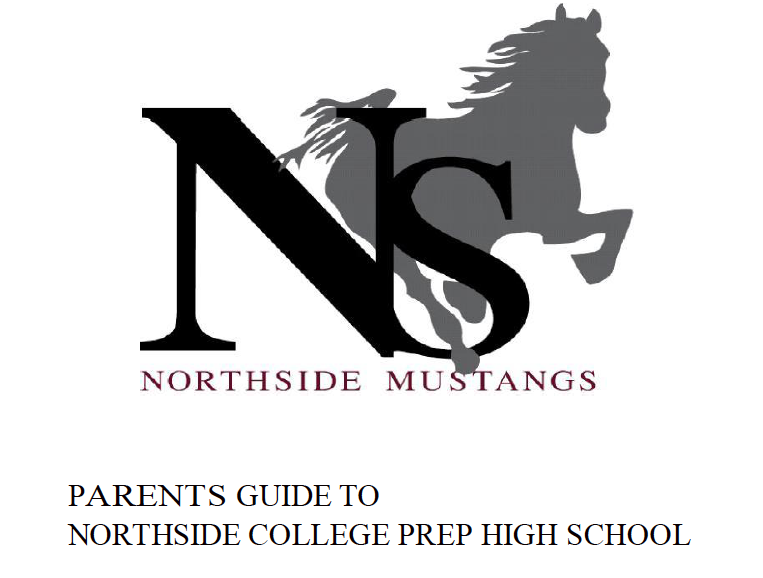# Navigating Northside

Navigating Northside, our parent handbook, is available on the school website under the Parents tab. Click HERE to access it directly.

 table div table+table+table+table+table+table+table+table+table+table+table+table+table+table+table+table+table+table+table+table+table+table+table+table+table+table+table+table+table+table+table+table+table+table+table+table+table+table+table+table+table+table div table{width:100%;padding:0}table div table+table+table+table+table+table+table+table+table+table+table+table+table+table+table+table+table+table+table+table+table+table+table+table+table+table+table+table+table+table+table+table+table+table+table+table+table+table+table+table+table+table div table img{width:96.23%;padding:0;float:none}table div table+table+table+table+table+table+table+table+table+table+table+table+table+table+table+table+table+table+table+table+table+table+table+table+table+table+table+table+table+table+table+table+table+table+table+table+table+table+table+table+table+table div table td{width:100%;padding:0 1.88% 18px}/* styles */# Congratulations NCP Varsity Women's Cross Country

At the Pat Savage Invitational meet hosted by Niles West HS
on Saturday, Sep. 29th, three of our Varsity Girls earned medals!

Congratulations to Erin Franke, (senior) who earned 12th place, Elia Ton-That (freshman), who earned 15th place, and Gwen Casey (junior) who earned 21st place.

# NCP Women's Tennis Advances in the City Tournament and to State Sectionals

Congratulations to Jennifer Labak for advancing to the next level in the City tournament.

Good luck to Jennifer Labak, Isabella McLaughlin, Tanvi Bagal, Hannah Kaczar, Audrey McManus, and Georgia Bates who will be playing in the State Sectionals at Nile's West on Friday, October 12.

 table div table+table+table+table+table+table+table+table+table+table+table+table+table+table+table+table+table+table+table+table+table+table+table+table+table+table+table+table+table+table+table+table+table+table+table+table+table+table+table+table+table+table+table+table+table+table div table{width:100%;padding:0}table div table+table+table+table+table+table+table+table+table+table+table+table+table+table+table+table+table+table+table+table+table+table+table+table+table+table+table+table+table+table+table+table+table+table+table+table+table+table+table+table+table+table+table+table+table+table div table img{width:96.23%;padding:0;float:none}table div table+table+table+table+table+table+table+table+table+table+table+table+table+table+table+table+table+table+table+table+table+table+table+table+table+table+table+table+table+table+table+table+table+table+table+table+table+table+table+table+table+table+table+table+table+table div table td{width:100%;padding:0 1.88% 18px}/* styles */# Yale Information Sessions

An Associate Director of Undergraduate Admissions will discuss academic programs, residential colleges and campus life, admissions, and financial aid. Free to attend and all are welcome. Registration is recommended but not required.

Tuesday, October 9, 06:30 - 08:00 pm
Whitney M. Young Magnet High School
211 S Laflin St, Chicago
Register HERE

# 100 Black Men of Chicago, Inc.'s - 16th Annual College Scholarship Fair

Saturday, October 13, 9:00 am - 2:00 pm
University of Illinois, at Chicago - Pavilion
525 S Racine Ave

Since 2003, the 100BMC has hosted an annual College Scholarship Fair with up to 200 Colleges and Universities interacting with nearly 5000 students, parents and educational partners.

Students can register HERE.

# Tufts University Admissions: Who Gets In and Why

Sunday, October 14, 02:00 - 04:00 pm
DoubleTree by Hilton Hotel Chicago - North Shore Conference Center
9599 Skokie Blvd, Skokie

The program will highlight the following:
1. Academics and campus life at Tufts University
2. The different components of a college application and how those materials are evaluated by selective admissions offices
3. Tips on successful essay writing and the impact of an application supplement
4. The role of recommendations and interviews in the process
5. A case study designed to resemble the admissions process, featuring five real applications from prior Tufts applicants

Students can register HERE for the workshop.

# Upcoming College Visits at Northside

Tuesday, October 9
Franklin University Switzerland, 9:48 am
Wellesley College, 9:48 am
West Virginia University, 9:48 am
Southern Illinois University, Edwardsville, 10:38 am
West Virginia University, 10:38 am
Berry College, 12:26 pm
Calvin College, 3:15 pm

Wednesday, October 10
Denison University, 9:48 am
Saint Michael's College, 9:48 am
Sarah Lawrence College, 9:48 am
Wesleyan University, 9:48 am
Yale University, 9:48 am
Macalester College, 12:00 pm
Amherst College, 12:26 pm
Swarthmore College, 3:15 pm
Washington University in St. Louis, 3:15 pm

Thursday, October 11
Coe College, 9:48 am
Marquette University, 9:48 am
Saint Louis University, 9:48 am
University of Utah, 9:48 am
Transylvania University, 10:38 am
Middlebury College, 12:26 pm
Northwestern University, 3:15 pm
Williams College, 3:15 pm

Friday, October 12
Santa Clara University, 9:48 am
Haverford College, 10:38 am
Adelphi University, 3:15 pm

Monday, October 15
Drexel University, 9:48 am
University of Miami, 9:48 am
California College of the Arts (San Francisco) , 11:36 am
Miami University, Oxford, 11:36 am
St. Lawrence University, 12:26 pm
University of Cincinnati, 3:15 pm

Tuesday, October 16
DePaul University, 9:48 am
Maryland Institute College of Art, 9:48 am
Pacific Northwest College of Art, 10:38 am
Elon University, 11:36 am
Eckerd College, 12:26 pm
Tufts University, 3:15 pm

Wednesday, October 17
Agnes Scott College, 10:31 am
University of Alabama at Birmingham (UAB), 12:13 pm
Binghamton University, 3:15 pm

Thursday, October 18
Occidental College, 9:48 am
Colby College, 12:26 pm
Mount Holyoke College, 12:26 pm
Illinois Wesleyan University, 3:15 pm

Friday, October 19
Worcester Polytechnic Institute, 9:48 am
Fairfield University, 10:38 am
Albion College, 11:36 am
Columbia University, 12:26 pm
Lewis & Clark College, 12:26 pm
Harvey Mudd College, 3:15 pm

The list of college visits is updated frequently. For the most current information, parents of juniors and seniors should log into their parent account for Naviance.

 table div table+table+table+table+table+table+table+table+table+table+table+table+table+table+table+table+table+table+table+table+table+table+table+table+table+table+table+table+table+table+table+table+table+table+table+table+table+table+table+table+table+table+table+table+table+table+table+table+table+table+table+table div table{width:100%;padding:0}table div table+table+table+table+table+table+table+table+table+table+table+table+table+table+table+table+table+table+table+table+table+table+table+table+table+table+table+table+table+table+table+table+table+table+table+table+table+table+table+table+table+table+table+table+table+table+table+table+table+table+table+table div table img{width:96.23%;padding:0;float:none}table div table+table+table+table+table+table+table+table+table+table+table+table+table+table+table+table+table+table+table+table+table+table+table+table+table+table+table+table+table+table+table+table+table+table+table+table+table+table+table+table+table+table+table+table+table+table+table+table+table+table+table+table div table td{width:100%;padding:0 1.88% 18px}/* styles */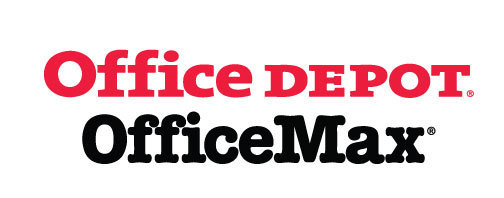# Give Back to Northside

Purchase school and office supplies at Office Depot and OfficeMax, and Northside can receive 5% back in credits or FREE supplies. Simply provide our school ID 70031171 at checkout, online or in stores. There’s no cost to you!

 table div table+table+table+table+table+table+table+table+table+table+table+table+table+table+table+table+table+table+table+table+table+table+table+table+table+table+table+table+table+table+table+table+table+table+table+table+table+table+table+table+table+table+table+table+table+table+table+table+table+table+table+table+table+table+table div table{width:100%;padding:0}table div table+table+table+table+table+table+table+table+table+table+table+table+table+table+table+table+table+table+table+table+table+table+table+table+table+table+table+table+table+table+table+table+table+table+table+table+table+table+table+table+table+table+table+table+table+table+table+table+table+table+table+table+table+table+table div table img{width:96.23%;padding:0;float:none}table div table+table+table+table+table+table+table+table+table+table+table+table+table+table+table+table+table+table+table+table+table+table+table+table+table+table+table+table+table+table+table+table+table+table+table+table+table+table+table+table+table+table+table+table+table+table+table+table+table+table+table+table+table+table+table div table td{width:100%;padding:0 1.88% 18px}/* styles */table.module-55{width:66.42%;padding:0}table div table+table+table+table+table+table+table+table+table+table+table+table+table+table+table+table+table+table+table+table+table+table+table+table+table+table+table+table+table+table+table+table+table+table+table+table+table+table+table+table+table+table+table+table+table+table+table+table+table+table+table+table+table+table+table+table div table{width:66.42%;float:none;margin-left:auto;margin-right:auto;padding:0}table div table+table+table+table+table+table+table+table+table+table+table+table+table+table+table+table+table+table+table+table+table+table+table+table+table+table+table+table+table+table+table+table+table+table+table+table+table+table+table+table+table+table+table+table+table+table+table+table+table+table+table+table+table+table+table+table div table a{border:0 none;text-decoration:none}table div table+table+table+table+table+table+table+table+table+table+table+table+table+table+table+table+table+table+table+table+table+table+table+table+table+table+table+table+table+table+table+table+table+table+table+table+table+table+table+table+table+table+table+table+table+table+table+table+table+table+table+table+table+table+table+table div table img{width:100%!important;border:0 none;text-decoration:none}table div table+table+table+table+table+table+table+table+table+table+table+table+table+table+table+table+table+table+table+table+table+table+table+table+table+table+table+table+table+table+table+table+table+table+table+table+table+table+table+table+table+table+table+table+table+table+table+table+table+table+table+table+table+table+table+table div table td{width:100%;padding:0}/* styles */

# Support Northside - Shop AmazonSmile

AmazonSmile is a simple and automatic way to support Northside every time you shop.

Please note that our AmazonSmile name is Friends of "North" "Side" (North Side - two words at AmazonSmile).

 table div table+table+table+table+table+table+table+table+table+table+table+table+table+table+table+table+table+table+table+table+table+table+table+table+table+table+table+table+table+table+table+table+table+table+table+table+table+table+table+table+table+table+table+table+table+table+table+table+table+table+table+table+table+table+table+table+table+table div table{width:100%;padding:0}table div table+table+table+table+table+table+table+table+table+table+table+table+table+table+table+table+table+table+table+table+table+table+table+table+table+table+table+table+table+table+table+table+table+table+table+table+table+table+table+table+table+table+table+table+table+table+table+table+table+table+table+table+table+table+table+table+table+table div table img{width:96.23%;padding:0;float:none}table div table+table+table+table+table+table+table+table+table+table+table+table+table+table+table+table+table+table+table+table+table+table+table+table+table+table+table+table+table+table+table+table+table+table+table+table+table+table+table+table+table+table+table+table+table+table+table+table+table+table+table+table+table+table+table+table+table+table div table td{width:100%;padding:0 1.88% 18px}/* styles */# Follow Us on Social Media

▪ NCP's Official Facebook Page
▪ Northside Prep Parents Facebook Page, a closed group for parents, by parents, to share their knowledge of Northside
▪ NCP's Twitter Page
▪ Friends of Northside Facebook Page, for news everyone can view
 ▪ Northside Prep Parents Facebook Page, a closed group for parents, by parents, to share their knowledge of Northside
 ▪ Friends of Northside Facebook Page, for news everyone can view
 table div table+table+table+table+table+table+table+table+table+table+table+table+table+table+table+table+table+table+table+table+table+table+table+table+table+table+table+table+table+table+table+table+table+table+table+table+table+table+table+table+table+table+table+table+table+table+table+table+table+table+table+table+table+table+table+table+table+table+table+table div table{width:100%;padding:0}table div table+table+table+table+table+table+table+table+table+table+table+table+table+table+table+table+table+table+table+table+table+table+table+table+table+table+table+table+table+table+table+table+table+table+table+table+table+table+table+table+table+table+table+table+table+table+table+table+table+table+table+table+table+table+table+table+table+table+table+table div table img{width:96.23%;padding:0;float:none}table div table+table+table+table+table+table+table+table+table+table+table+table+table+table+table+table+table+table+table+table+table+table+table+table+table+table+table+table+table+table+table+table+table+table+table+table+table+table+table+table+table+table+table+table+table+table+table+table+table+table+table+table+table+table+table+table+table+table+table+table div table td{width:100%;padding:0 1.88% 18px}/* styles */Seniors, click HERE for the Class of 2019 web page.

Northside’s website can be found HERE.

Friends of Northside's website can be found HERE.

To see all volunteer opportunities on one page, click HERE.

To ensure delivery of This Week at Northside, please add Thisweek@friendsofnorthside.org to your address book.

Please do not reply to this e-mail. Send your questions or comments to Friends of Northside.

# Do you have news or team results to share with the Northside parent community?

 table div table+table+table+table+table+table+table+table+table+table+table+table+table+table+table+table+table+table+table+table+table+table+table+table+table+table+table+table+table+table+table+table+table+table+table+table+table+table+table+table+table+table+table+table+table+table+table+table+table+table+table+table+table+table+table+table+table+table+table+table+table+table div table{width:100%;padding:0}table div table+table+table+table+table+table+table+table+table+table+table+table+table+table+table+table+table+table+table+table+table+table+table+table+table+table+table+table+table+table+table+table+table+table+table+table+table+table+table+table+table+table+table+table+table+table+table+table+table+table+table+table+table+table+table+table+table+table+table+table+table+table div table img{width:96.23%;padding:0;float:none}table div table+table+table+table+table+table+table+table+table+table+table+table+table+table+table+table+table+table+table+table+table+table+table+table+table+table+table+table+table+table+table+table+table+table+table+table+table+table+table+table+table+table+table+table+table+table+table+table+table+table+table+table+table+table+table+table+table+table+table+table+table+table div table td{width:100%;padding:0 1.88% 18px}/* styles */